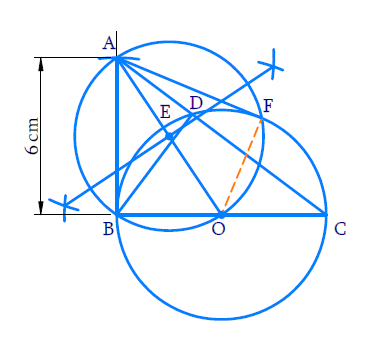# Ex.11.2 Q6 Constructions Solution - NCERT Maths Class 10

Go back to  'Ex.11.2'

## Question

Let $$ABC$$ be a right triangle in which $$AB = 6 \,\rm{cm},$$ $$BC = 8 \,\rm{cm}$$ and \begin{align}\angle \rm{B}=90^{\circ}.\end{align}  $$BD$$ is the perpendicular to $$AC$$. The circle through $$B$$, $$C$$ and $$D$$ is drawn. Construct the tangents from $$A$$ to this circle.

## Text Solution

#### Steps:Steps of construction:

(i) Draw \begin{align}\rm{BC}=8 \rm{cm}\end{align}. Draw the perpendicular at $$B$$ and cut \begin{align}\rm{BA}=6 \rm{cm}\end{align}on it. Join $$AC$$ right \begin{align}\triangle {ABC}\end{align}  is obtained.

(ii) Draw $$BD$$ perpendicular to $$AC$$.

(iii) Since \begin{align}\angle \rm{BDC}=90^{\circ}\end{align} and the circle has to pass through $$B, C$$ and $$D.$$ $$BC$$ must be a diameter of this circle. So, take $$O$$ as the midpoint of $$BC$$ and with $$O$$ as centre and $$OB$$ as radius draw a circle which will pass through $$B$$, $$C$$ and $$D$$.

(iv) To draw tangents from $$A$$ to the circle with center $$O$$.

(a) Join $$OA$$, and draw its perpendicular bisectors to intersect $$OA$$ at $$E$$.

(b) With $$E$$ as center and $$EA$$ as radius draw a circle which intersects the  previous circle at $$B$$ and $$F$$.

(c) Join $$AF$$.

$$AB$$ and $$AF$$ are the required tangents.

Proof:

$$\angle {{ABO}} = \angle {{AFO}} = 90^\circ$$  ( Angle in a semi $$-$$ circle)

$$\therefore$$ $${AB} \bot {OB}$$ and $${AF} \bot {OF}$$

Hence $$AB$$ and $$AF$$ are the tangents from $$A$$ to the circle with centre $$O$$.

Learn from the best math teachers and top your exams

• Live one on one classroom and doubt clearing
• Practice worksheets in and after class for conceptual clarity
• Personalized curriculum to keep up with school